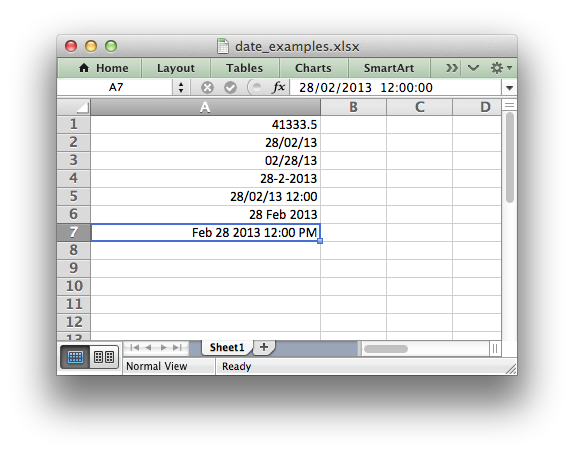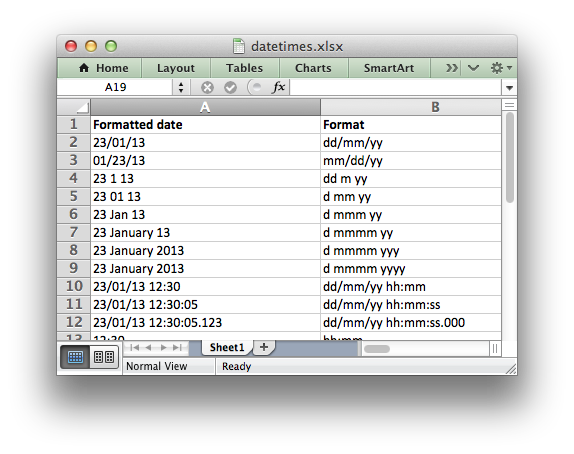# Working with Dates and Time

Dates and times in Excel are represented by real numbers, for example “Jan 1 2013 12:00 PM” is represented by the number 41275.5.

The integer part of the number stores the number of days since the epoch and the fractional part stores the percentage of the day.

A date or time in Excel is just like any other number. To display the number as a date you must apply an Excel number format to it. Here are some examples:

```import xlsxwriter

workbook = xlsxwriter.Workbook('date_examples.xlsx')

# Widen column A for extra visibility.
worksheet.set_column('A:A', 30)

# A number to convert to a date.
number = 41333.5

# Write it as a number without formatting.
worksheet.write('A1', number)                # 41333.5

worksheet.write('A2', number, format2)       # 28/02/13

worksheet.write('A3', number, format3)       # 02/28/13

worksheet.write('A4', number, format4)       # 28-2-2013

worksheet.write('A5', number, format5)       # 28/02/13 12:00

format6 = workbook.add_format({'num_format': 'd mmm yyyy'})
worksheet.write('A6', number, format6)       # 28 Feb 2013

format7 = workbook.add_format({'num_format': 'mmm d yyyy hh:mm AM/PM'})
worksheet.write('A7', number, format7)       # Feb 28 2013 12:00 PM

workbook.close()
```To make working with dates and times a little easier the XlsxWriter module provides a `write_datetime()` method to write dates in standard library `datetime` format.

Specifically it supports datetime objects of type `datetime.datetime`, `datetime.date`, `datetime.time` and `datetime.timedelta`.

There are many way to create datetime objects, for example the `datetime.datetime.strptime()` method:

```date_time = datetime.datetime.strptime('2013-01-23', '%Y-%m-%d')
```

See the `datetime` documentation for other date/time creation methods.

As explained above you also need to create and apply a number format to format the date/time:

```date_format = workbook.add_format({'num_format': 'd mmmm yyyy'})
worksheet.write_datetime('A1', date_time, date_format)

# Displays "23 January 2013"
```

Here is a longer example that displays the same date in a several different formats:

```from datetime import datetime
import xlsxwriter

# Create a workbook and add a worksheet.
workbook = xlsxwriter.Workbook('datetimes.xlsx')

# Expand the first columns so that the dates are visible.
worksheet.set_column('A:B', 30)

worksheet.write('A1', 'Formatted date', bold)
worksheet.write('B1', 'Format', bold)

# Create a datetime object to use in the examples.

date_time = datetime.strptime('2013-01-23 12:30:05.123',
'%Y-%m-%d %H:%M:%S.%f')

# Examples date and time formats.
date_formats = (
'dd/mm/yy',
'mm/dd/yy',
'dd m yy',
'd mm yy',
'd mmm yy',
'd mmmm yy',
'd mmmm yyy',
'd mmmm yyyy',
'dd/mm/yy hh:mm',
'dd/mm/yy hh:mm:ss',
'dd/mm/yy hh:mm:ss.000',
'hh:mm',
'hh:mm:ss',
'hh:mm:ss.000',
)

# Start from first row after headers.
row = 1

# Write the same date and time using each of the above formats.
for date_format_str in date_formats:

# Create a format for the date or time.
'align': 'left'})

# Write the same date using different formats.
worksheet.write_datetime(row, 0, date_time, date_format)

# Also write the format string for comparison.
worksheet.write_string(row, 1, date_format_str)

row += 1

workbook.close()
```## Default Date Formatting

In certain circumstances you may wish to apply a default date format when writing datetime objects, for example, when handling a row of data with `write_row()`.

In these cases it is possible to specify a default date format string using the `Workbook()` constructor `default_date_format` option:

```workbook = xlsxwriter.Workbook('datetimes.xlsx', {'default_date_format':
'dd/mm/yy'})
date_time = datetime.now()
worksheet.write_datetime(0, 0, date_time)  # Formatted as 'dd/mm/yy'

workbook.close()
```

## Timezone Handling

Excel doesn’t support timezones in datetimes/times so there isn’t any fail-safe way that XlsxWriter can map a Python timezone aware datetime into an Excel datetime. As such the user should handle the timezones in some way that makes sense according to their requirements. Usually this will require some conversion to a timezone adjusted time and the removal of the `tzinfo` from the datetime object so that it can be passed to `write_datetime()`:

```utc_datetime = datetime(2016, 9, 23, 14, 13, 21, tzinfo=utc)
naive_datetime = utc_datetime.replace(tzinfo=None)

worksheet.write_datetime(row, 0, naive_datetime, date_format)
```

Alternatively the `Workbook()` constructor option `remove_timezone` can be used to strip the timezone from datetime values passed to `write_datetime()`. The default is `False`. To enable this option use:

```workbook = xlsxwriter.Workbook(filename, {'remove_timezone': True})
```

When Working with Pandas and XlsxWriter you can pass the argument as follows:

```writer = pd.ExcelWriter('pandas_example.xlsx',
engine='xlsxwriter',
options={'remove_timezone': True})
```